# Difference between revisions of "Path integral formulation"

The path integral formulation, here from the statistical mechanical point of view, is an elegant method by which quantum mechanical contributions can be incorporated within a classical simulation using Feynman path integrals (see the additional reading section). Such simulations are particularly applicable to light atoms and molecules such as hydrogen, helium, neon and argon, as well as quantum rotators such as methane and hydrogen-bonded systems such as water. From a more idealised point of view path integrals are often used to study quantum hard spheres.

## Principles

In the path integral formulation the canonical partition function (in one dimension) is written as ( Eq. 1)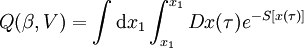$Q(\beta, V)= \int {\mathrm d} x_1 \int_{x_1}^{x_1} Dx(\tau)e^{-S[x(\tau)]}$

where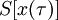$S[x(\tau)]$ is the Euclidean action, given by ( Eq. 2)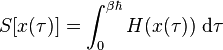$S[x(\tau)] = \int_0^{\beta \hbar} H(x(\tau)) ~{\mathrm d}\tau$

where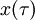$x(\tau)$ is the path in time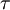$\tau$ and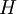$H$ is the Hamiltonian. This leads to ( Eq. 3)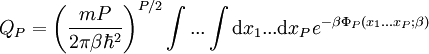$Q_P = \left( \frac{mP}{2 \pi \beta \hbar^2} \right)^{P/2} \int ... \int {\mathrm d}x_1... {\mathrm d}x_P e^{-\beta \Phi_P (x_1...x_P;\beta)}$

where the Euclidean time is discretised in units of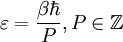$\varepsilon = \frac{\beta \hbar}{P}, P \in {\mathbb Z}$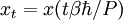$x_t = x(t \beta \hbar/P)$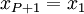$x_{P+1}=x_1$

and ( Eq. 4)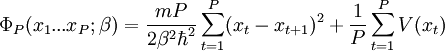$\Phi_P (x_1...x_P;\beta)= \frac{mP}{2\beta^2 \hbar^2} \sum_{t=1}^P (x_t - x_{t+1})^2 + \frac{1}{P} \sum_{t=1}^P V(x_t)$

where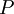$P$ is the Trotter number. In the Trotter limit, where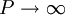$P \rightarrow \infty$ these equations become exact. In the case where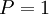$P=1$ these equations revert to a classical simulation. It has long been recognised that there is an isomorphism between this discretised quantum mechanical description, and the classical statistical mechanics of polyatomic fluids, in particular flexible ring molecules, due to the periodic boundary conditions in imaginary time. It can be seen from the first term of the above equation that each particle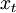$x_t$ interacts with is neighbours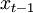$x_{t-1}$ and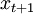$x_{t+1}$ via a harmonic spring. The second term provides the internal potential energy.

The following is a schematic for the interaction between atom$i$ (green) and atom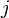$j$ (orange). Here we show the atoms having five Trotter slices (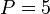$P=5$), forming what can be thought of as a "ring polymer molecule". The harmonic springs between Trotter slices are in yellow, and white/blue bonds represent the classical intermolecular pair potential.

In three dimensions one has the density operator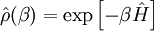$\hat{\rho} (\beta) = \exp\left[ -\beta \hat{H} \right]$

which thanks to the Trotter formula we can tease out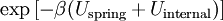$\exp \left[ -\beta (U_{\mathrm {spring}}+ U_{\mathrm{internal}} ) \right]$, where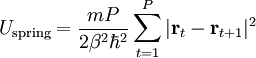$U_{\mathrm {spring}} = \frac{mP}{2\beta^2 \hbar^2} \sum_{t=1}^P | \mathbf{r}_t - \mathbf{r}_{t+1} |^2$

and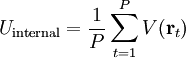$U_{\mathrm{internal}}= \frac{1}{P} \sum_{t=1}^P V(\mathbf{r}_t)$

The internal energy is given by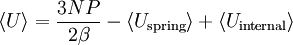$\langle U \rangle = \frac{3NP}{2\beta}- \langle U_{\mathrm {spring}} \rangle + \langle U_{\mathrm{internal}} \rangle$

The average kinetic energy is known as the primitive estimator, i.e.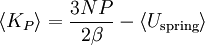$\langle K_P \rangle = \frac{3NP}{2\beta}- \langle U_{\mathrm {spring}} \rangle$

## Harmonic oscillator

The density matrix for a harmonic oscillator is given by ( Eq. 10-44)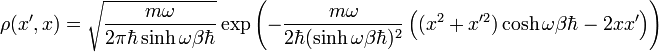$\rho(x',x)= \sqrt{ \frac{m \omega}{2 \pi \hbar \sinh \omega \beta \hbar} } \exp \left( - \frac{m \omega}{2 \hbar (\sinh \omega \beta \hbar)^2 } \left( (x^2 + x'^2 ) \cosh \omega \beta \hbar - 2xx'\right)\right)$

## Wick rotation and imaginary time

Wick rotation . One can identify the inverse temperature,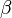$\beta$ with an imaginary time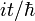$it/\hbar$ (see  § 2.4).

## Rotational degrees of freedom

In the case of systems having (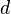$d$) rotational degrees of freedom the Hamiltonian can be written in the form ( Eq. 2.1):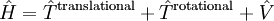$\hat{H} = \hat{T}^{\mathrm {translational}} + \hat{T}^{\mathrm {rotational}}+ \hat{V}$

where the rotational part of the kinetic energy operator is given by ( Eq. 2.2)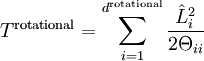$T^{\mathrm {rotational}} = \sum_{i=1}^{d^{\mathrm {rotational}}} \frac{\hat{L}_i^2}{2\Theta_{ii}}$

where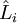$\hat{L}_i$ are the components of the angular momentum operator, and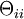$\Theta_{ii}$ are the moments of inertia.

## Rigid rotators

Main article: Rigid top propagator

## Computer simulation techniques

The following are a number of commonly used computer simulation techniques that make use of the path integral formulation applied to phases of condensed matter

#### Path integral Monte Carlo

Path integral Monte Carlo (PIMC) 

#### Path integral molecular dynamics

Path integral molecular dynamics (PIMD) 

#### Centroid molecular dynamics

Centroid molecular dynamics (CMD)     

#### Ring polymer molecular dynamics

Ring polymer molecular dynamics (RPMD)   (Contraction scheme  )

#### Grand canonical Monte Carlo

A path integral version of the Widom test-particle method for grand canonical Monte Carlo simulations: